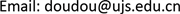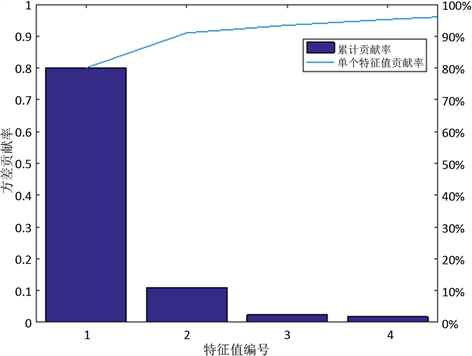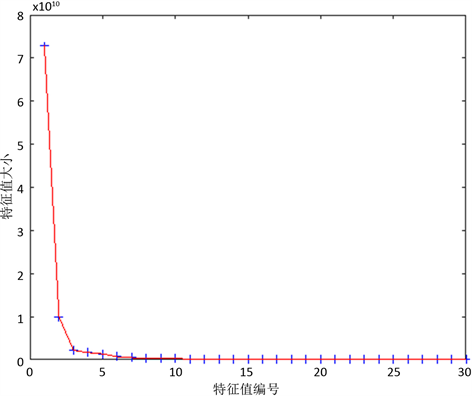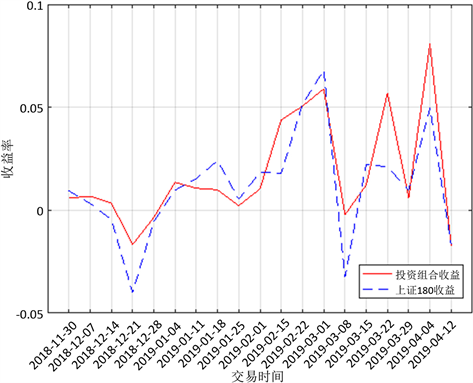﻿ 基于EKPCA算法的多因子选股模型研究 Research on Multi-Factor Stock Selection Model Based on EKPCA Algorithm

Finance
Vol. 09  No. 04 ( 2019 ), Article ID: 31245 , 14 pages
10.12677/FIN.2019.94041

Research on Multi-Factor Stock Selection Model Based on EKPCA Algorithm

Yu Huang, Houqing Fang, Lingfei Dai, Tingting Chen

Faculty of Science, Jiangsu University, Zhenjiang JiangsuReceived: Jun. 20th, 2019; accepted: Jul. 3rd, 2019; published: Jul. 12th, 2019ABSTRACT

The multi-factor stock selection model is the mainstream method in quantitative investment. This paper introduces the Efficient Kernel Principal Component Analysis (EKPCA) algorithm for the first time. The high-efficiency kernel principal component is used as the independent variable to establish the regression equation to predict the rate of return and construct a multi-factor stock selection model. Based on the empirical analysis of the constituents of SSE 180, this paper selects more than 50 impact factors including fundamentals, technical indicators and investor sentiment indicators, and uses the EKPCA algorithm to determine the basic model and extracts high-efficiency kernel principal components in the high-dimensional feature space. Compared with the classical KPCA algorithm, the EKPCA algorithm has higher feature extraction efficiency. The backtest results show that the beta coefficient and Sharpe ratio of the constructed portfolio are better than the market benchmark level in the selected time period, which indicates that the model has a better stock picking effect.

Keywords:EKPCA Algorithm, Multi-Factor Stock Selection, The General Entropy, Feature Extraction, Kernel Function1. 引言

2. 影响因子选取

3. 多重共线性检验

$\text{VIF}=\frac{1}{1-{R}^{2}}$

4. 基于EKPCA算法的多因子选股模型建立

4.1. EKPCA算法的基本原理

${K}_{ij}=〈\Phi \left({x}_{i}\right),\Phi \left({x}_{j}\right)〉=\Phi {\left({x}_{i}\right)}^{\text{T}}\Phi \left({x}_{j}\right)=\kappa \left({x}_{i},{x}_{j}\right),\text{\hspace{0.17em}}i=1,2,\cdots ,N;\text{\hspace{0.17em}}j=1,2,\cdots ,N$ (1)

EKPCA算法与KPCA算法相比主要有两个优点：第一，基本模式的个数一般远远小于全体训练样本的个数，这使得EKPCA算法比KPCA算法的抽取效率高；第二，在特征抽取时，KPCA需要对 $N×N$ (N是全体训练样本的个数)的核矩阵进行特征分解，而EKPCA只需要对 $s×s$ (s是基本模式的个数)的核矩阵进行特征分解，这说明EKPCA比KPCA需要更少的存储空间  。

4.2. 核函数参数选择

${c}_{ij}=\frac{\Phi {\left({x}_{i}\right)}^{\text{T}}\Phi \left({x}_{j}\right)}{‖\Phi \left({x}_{i}\right)‖\cdot ‖\Phi \left({x}_{j}\right)‖},\text{\hspace{0.17em}}\text{\hspace{0.17em}}i=1,2,\cdots ,N;\text{\hspace{0.17em}}j=1,2,\cdots ,N$ (2)

${c}_{ij}=\frac{\kappa \left({x}_{i},{x}_{j}\right)}{‖\kappa \left({x}_{i},{x}_{i}\right)‖\cdot ‖\kappa \left({x}_{j},{x}_{j}\right)‖},\text{\hspace{0.17em}}i=1,2,\cdots ,N;\text{\hspace{0.17em}}j=1,2,\cdots ,N$ (3)

${c}_{ij}=\kappa \left({x}_{i},{x}_{j}\right)$ (4)

$\text{Entro}\left({c}_{ij}\right)=-{\sum }_{i=1}^{N}{\sum }_{j=1}^{N}|{c}_{ij}|\mathrm{log}|{c}_{ij}|$ (5)

$\text{Entro}\left({c}_{ij}\right)=-{\sum }_{i=1}^{N}{\sum }_{j=1}^{N}|\kappa \left({x}_{i},{x}_{j}\right)|\mathrm{log}|\kappa \left({x}_{i},{x}_{j}\right)|$ (6)

$\text{Max}\text{\hspace{0.17em}}\text{Entro}\left({c}_{ij}\right)$

$\text{s}.\text{t}.\text{\hspace{0.17em}}\left\{\begin{array}{l}0.1\le c\le 10.0\\ 1\le d\le 10\\ d为整数\end{array}$

$1.0\le \theta \le 10.0$

4.3. 确定基本模式

$\mathrm{cos}\left({v}_{i},{v}_{j}\right)=\frac{\left({v}_{i},{v}_{j}\right)}{‖{v}_{i}‖\cdot ‖{v}_{j}‖}$

$\mathrm{cos}\left({v}_{m},{v}_{n}\right)={\mathrm{min}}_{1\le i,j\le N}\mathrm{cos}\left({v}_{i},{v}_{j}\right)\left(1\le m,n\le N\right)$ (7)

${K}_{t-1}=\left[\begin{array}{ccc}k\left({x}_{1},{{x}^{\prime }}_{1}\right)& \cdots & k\left({x}_{1},{{x}^{\prime }}_{t-1}\right)\\ k\left({x}_{2},{{x}^{\prime }}_{1}\right)& \cdots & k\left({x}_{2},{{x}^{\prime }}_{t-1}\right)\\ ⋮& \ddots & ⋮\\ k\left({x}_{N},{{x}^{\prime }}_{1}\right)& \cdots & k\left({x}_{N},{{x}^{\prime }}_{t-1}\right)\end{array}\right]$

${v}_{p}={\left(k\left({x}_{1},{x}_{p}\right),k\left({x}_{2},{x}_{p}\right),\cdots ,k\left({x}_{N},{x}_{p}\right)\right)}^{\text{T}}.$

$\text{cosdis}\left({v}_{p},{K}_{t-1}\right)=\frac{1}{t-1}{\sum }_{q=1}^{t-1}\text{cos}\left({v}_{p},{v}_{q}\right)$ (8)

4.4. 重建KPCA模型

$\stackrel{¯}{\Phi }=\frac{1}{N}\underset{i=1}{\overset{N}{\sum }}\Phi \left({x}_{i}\right)=0$

$\begin{array}{c}{\sigma }^{2}=\frac{1}{N}\underset{i=1}{\overset{N}{\sum }}{\left({v}^{\text{T}}{x}_{i}-\mu \right)}^{2}=\frac{1}{N}\underset{i=1}{\overset{N}{\sum }}{\left({v}^{\text{T}}{x}_{i}\right)}^{2}=\frac{1}{N}\underset{i=1}{\overset{N}{\sum }}\left({v}^{\text{T}}{x}_{i}\right){\left({v}^{\text{T}}{x}_{i}\right)}^{\text{T}}\\ =\frac{1}{N}{\sum }_{i=1}^{N}{v}^{\text{T}}{x}_{i}{x}_{i}^{\text{T}}v={v}^{\text{T}}\left(\frac{1}{N}{\sum }_{i=1}^{N}{x}_{i}{x}_{i}^{\text{T}}\right)v={v}^{\text{T}}Cv\end{array}$ (9)

$v=\mathrm{arg}\mathrm{max}{v}^{\text{T}}Cv$ (10)

$\text{s}.\text{t}.\text{\hspace{0.17em}}\text{\hspace{0.17em}}‖v‖=1$

$f\left(v,\lambda \right)={v}^{\text{T}}Cv-\lambda \left({v}^{\text{T}}v-1\right)$ (11)

$f\left(v,\lambda \right)$ 对v和 $\lambda$ 的偏导数为零得

$Cv=\lambda v$ (12)

${v}^{\text{T}}v=1$ (13)

$v=\mathrm{arg}\mathrm{max}\lambda$ (14)$\lambda \alpha =D\alpha$ (15)

$\begin{array}{c}{K}_{2}={\left[\begin{array}{ccc}k\left({x}_{1},{{x}^{\prime }}_{1}\right)& \cdots & k\left({x}_{1},{{x}^{\prime }}_{s}\right)\\ k\left({x}_{2},{{x}^{\prime }}_{1}\right)& \cdots & k\left({x}_{2},{{x}^{\prime }}_{s}\right)\\ ⋮& \ddots & ⋮\\ k\left({x}_{s},{{x}^{\prime }}_{1}\right)& \cdots & k\left({x}_{s},{{x}^{\prime }}_{s}\right)\end{array}\right]}_{s×s}=\left[\begin{array}{ccc}\Phi {\left({{x}^{\prime }}_{1}\right)}^{\text{T}}\Phi \left({{x}^{\prime }}_{1}\right)& \cdots & \Phi {\left({{x}^{\prime }}_{1}\right)}^{\text{T}}\Phi \left({{x}^{\prime }}_{s}\right)\\ \Phi {\left({{x}^{\prime }}_{2}\right)}^{\text{T}}\Phi \left({{x}^{\prime }}_{s}\right)& \cdots & \Phi {\left({{x}^{\prime }}_{1}\right)}^{\text{T}}\Phi \left({{x}^{\prime }}_{s}\right)\\ ⋮& \ddots & ⋮\\ \Phi {\left({{x}^{\prime }}_{s}\right)}^{\text{T}}\Phi \left({{x}^{\prime }}_{s}\right)& \cdots & \Phi {\left({{x}^{\prime }}_{1}\right)}^{\text{T}}\Phi \left({{x}^{\prime }}_{s}\right)\end{array}\right]\\ =\left[\begin{array}{c}\Phi {\left({{x}^{\prime }}_{1}\right)}^{\text{T}}\\ ⋮\\ \Phi {\left({{x}^{\prime }}_{s}\right)}^{\text{T}}\end{array}\right]\left[\begin{array}{ccc}\Phi \left({{x}^{\prime }}_{1}\right)& \cdots & \Phi \left({{x}^{\prime }}_{s}\right)\end{array}\right]\end{array}$ (16)

$D=\frac{1}{N}\Phi \left({x}_{j}\right)\Phi {\left({x}_{j}\right)}^{\text{T}},$ (17)

${K}_{1}={\left({K}_{s}\right)}^{\text{T}}=k\left({{x}^{\prime }}_{i},{x}_{j}\right)=\Phi {\left({{x}^{\prime }}_{i}\right)}^{\text{T}}\Phi \left({x}_{j}\right),$ (18)

${K}_{2}=\Phi {\left({{x}^{\prime }}_{i}\right)}^{\text{T}}\Phi \left({{x}^{\prime }}_{r}\right),$ (19)

$\lambda \alpha =\frac{1}{N}\Phi \left({x}_{j}\right)\Phi {\left({x}_{j}\right)}^{\text{T}}\alpha$ (20)

$\lambda \left(\Phi {\left({{x}^{\prime }}_{i}\right)}^{\text{T}}\Phi \left({{x}^{\prime }}_{r}\right)\right)\beta =\frac{1}{N}\left(\Phi {\left({{x}^{\prime }}_{i}\right)}^{\text{T}}\Phi \left({x}_{j}\right)\right)\left(\Phi {\left({x}_{j}\right)}^{\text{T}}\Phi \left({{x}^{\prime }}_{i}\right)\right)\beta$ (21)

$\lambda {K}_{2}\beta =\frac{1}{N}{K}_{1}{\left({K}_{1}\right)}^{\text{T}}\beta$ (22)

$B={\left[\frac{{\sum }_{i=1}^{s}{\beta }_{1i}^{\ast }k\left({{x}^{\prime }}_{i},x\right)}{\sqrt{{\lambda }_{1}}},\frac{{\sum }_{i=1}^{s}{\beta }_{2i}^{\ast }k\left({{x}^{\prime }}_{i},x\right)}{\sqrt{{\lambda }_{2}}},\cdots ,\frac{{\sum }_{i=1}^{s}{\beta }_{pi}^{\ast }k\left({{x}^{\prime }}_{i},x\right)}{\sqrt{{\lambda }_{p}}}\right]}^{\text{T}}$ (23)

4.5. 多元线性回归预测

$y={\sum }_{k=1}^{p}{\omega }_{k}{B}_{k}+\epsilon$ (24)

$\stackrel{^}{\omega }={\left({B}^{\text{T}}B\right)}^{-1}{B}^{\text{T}}y$ (25)

$\stackrel{^}{y}=\underset{k=1}{\overset{p}{\sum }}{\stackrel{^}{\omega }}_{k}{B}_{k}$

5. 实证分析及实验结果Figure 1. Variance contribution histogram and cumulative contribution histogramFigure 2. Eigenvalue lithotripsy

$y=\frac{{p}_{1}-{p}_{0}}{{p}_{0}}$Table 4. Parameter estimation of multiple linear regression

6. 模型评价Figure 3. Portfolio performance analysis

7. 总结

Research on Multi-Factor Stock Selection Model Based on EKPCA Algorithm[J]. 金融, 2019, 09(04): 327-340. https://doi.org/10.12677/FIN.2019.94041

1. 1. 王春丽, 刘光, 王齐. 多因子量化选股模型与择时策略[J]. 东北财经大学学报, 2018, 119(5): 83-89.

2. 2. 范烨. 多因子选股模型建立的研究[J]. 全国流通经济, 2018(3): 64-65.

3. 3. 李娜, 毛国君, 邓康立. 基于k-means聚类的股票KDJ类指标综合分析方法[J]. 计算机与现代化, 2018, 278(10): 12-17.

4. 4. 苏治, 傅晓媛. 核主成分遗传算法与SVR选股模型改进[J]. 统计研究, 2013, 30(5): 54-62.

5. 5. 贾秀娟. 基于随机森林的支持向量机量化选股[J]. 区域金融研究, 2019(1): 27-30.

6. 6. 吕凯晨, 闫宏飞, 陈翀. 基于沪深300成分股的量化投资策略研究[J]. 广西师范大学学报(自然科学版), 2019, 37(1): 1-12.

7. 7. 徐景昭. 基于多因子模型的量化选股分析[J]. 金融理论探索, 2017(3): 30-38.

8. 8. 朱晨曦. 我国A股市场多因子量化选股模型实证分析[D]: [硕士学位论文]. 北京: 首都经济贸易大学, 2017.

9. 9. 凌士勤, 苏乐. 投资者情绪与股票收益的实证研究——基于扩展卡尔曼滤波的方法[J]. 时代金融, 2017(6): 192.

10. 10. 王锐. 岭回归分析在解决经济数据共线性问题中的应用[J]. 经济研究导刊, 2018(22): 144-147.

11. 11. Fan, Z., Wang, J., Xu, B. and Tang, P. (2014) An Efficient KPCA Algorithm Based on Feature Correla-tion Evaluation. Neural Computing and Applications, 24, 1795-1806. https://doi.org/10.1007/s00521-013-1424-9

12. 12. 周志华. 机器学习[M]. 北京: 清华大学出版社, 2016: 60-62, 128.

13. 13. Sun, R., Tsung, F. and Qu, L. (2007) Evolving Kernel Principal Component Analysis for Fault Diagnosis. Computers & Industrial Engineering, 53, 361-371. https://doi.org/10.1016/j.cie.2007.06.029

14. 14. 范自柱. 新型特征抽取算法研究[M]. 合肥: 中国科学技术大学出版社, 2016: 95-102, 122-128.

15. 15. 吴世农, 韦绍永. 上海股市投资组合规模和风险关系的实证研究[J]. 经济研究, 1998(4): 22-29.